Mathematics
Easy

Question

#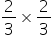=

##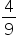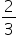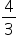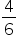Hint:

## The correct answer is:### Step by step solution:Required to find:The way to multiply a fraction is by simply multiplying straight across. The product is also supposed to be a fraction so the numerator of the product will be the product of the numerators of the given numbers and the denominator of the product will be the product of the denominators of the given numbers.Therefore, in the given question,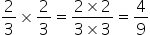Thus, the correct option is option (a)#### With Turito Foundation.#### Get an Expert Advice From Turito.